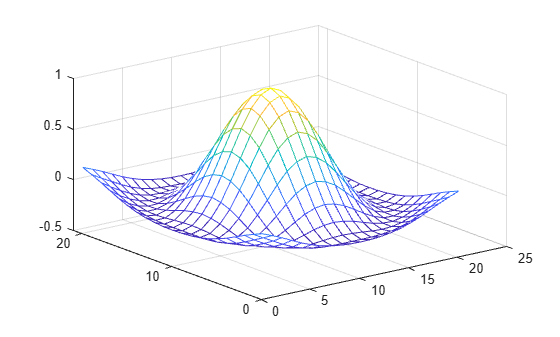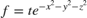interpn

Interpolation for 1-D, 2-D, 3-D, and N-D gridded data in ndgrid format

Description

example

Vq = interpn(X1,X2,...,Xn,V,Xq1,Xq2,...,Xqn) returns interpolated values of a function of n variables at specific query points using linear interpolation. The results always pass through the original sampling of the function. X1,X2,...,Xn contain the coordinates of the sample points. V contains the corresponding function values at each sample point. Xq1,Xq2,...,Xqn contain the coordinates of the query points.

Vq = interpn(V,Xq1,Xq2,...,Xqn) assumes a default grid of sample points. The default grid consists of the points, 1,2,3,...ni in each dimension. The value of ni is the length of the ith dimension in V. Use this syntax when you want to conserve memory and are not concerned about the absolute distances between points.

Vq = interpn(V) returns the interpolated values on a refined grid formed by dividing the interval between sample values once in each dimension.

example

Vq = interpn(V,k) returns the interpolated values on a refined grid formed by repeatedly halving the intervals k times in each dimension. This results in 2^k-1 interpolated points between sample values.

example

Vq = interpn(___,method) specifies an alternative interpolation method: 'linear', 'nearest', 'pchip','cubic', 'makima', or 'spline'. The default method is 'linear'.

example

Vq = interpn(___,method,extrapval) also specifies extrapval, a scalar value that is assigned to all queries that lie outside the domain of the sample points.

If you omit the extrapval argument for queries outside the domain of the sample points, then based on the method argument interpn returns one of the following:

• The extrapolated values for the 'spline' and 'makima' methods

• NaN values for other interpolation methods

Examples

collapse all

Define the sample points and values.

x = [1 2 3 4 5];
v = [12 16 31 10 6];

Define the query points, xq, and interpolate.

xq = (1:0.1:5);
vq = interpn(x,v,xq,'cubic');

Plot the result.

figure
plot(x,v,'o',xq,vq,'-');
legend('Samples','Cubic Interpolation');Create a set of grid points and corresponding sample values.

[X1,X2] = ndgrid((-5:1:5));
R = sqrt(X1.^2 + X2.^2)+ eps;
V = sin(R)./(R);

Interpolate over a finer grid using ntimes=1.

Vq = interpn(V,'cubic');
mesh(Vq);Create the grid vectors, x1, x2, and x3. These vectors define the points associated with the values in V.

x1 = 1:100;
x2 = (1:50)';
x3 = 1:30;

Define the sample values to be a 100-by-50-by-30 random number array, V. Use the gallery function to create the array.

rng('default')
V = rand(100,50,30);

Evaluate V at three points outside the domain of x1, x2, and x3. Specify extrapval = -1.

xq1 = [0 0 0];
xq2 = [0 0 51];
xq3 = [0 101 102];
vq = interpn(x1,x2,x3,V,xq1,xq2,xq3,'linear',-1)
vq = 1×3

-1    -1    -1

All three points evaluate to -1 because they are outside the domain of x1, x2, and x3.

Define an anonymous function that represents.

f = @(x,y,z,t) t.*exp(-x.^2 - y.^2 - z.^2);

Create a grid of points in. Then, pass the points through the function to create the sample values, V.

[x,y,z,t] = ndgrid(-1:0.2:1,-1:0.2:1,-1:0.2:1,0:2:10);
V = f(x,y,z,t);

Now, create the query grid.

[xq,yq,zq,tq] = ...
ndgrid(-1:0.05:1,-1:0.08:1,-1:0.05:1,0:0.5:10);

Interpolate V at the query points.

Vq = interpn(x,y,z,t,V,xq,yq,zq,tq);

Create a movie to show the results.

figure('renderer','zbuffer');
nframes = size(tq, 4);
for j = 1:nframes
slice(yq(:,:,:,j),xq(:,:,:,j),zq(:,:,:,j),...
Vq(:,:,:,j),0,0,0);
caxis([0 10]);
M(j) = getframe;
end
movie(M);Input Arguments

collapse all

Sample grid points, specified as real arrays or vectors. The sample grid points must be unique.

• If X1,X2,...,Xn are arrays, then they contain the coordinates of a full grid (in ndgrid format). Use the ndgrid function to create the X1,X2,...,Xn arrays together. These arrays must be the same size.

• If X1,X2,...,Xn are vectors, then they are treated as grid vectors. The values in these vectors must be strictly monotonic, either increasing or decreasing.

Example: [X1,X2,X3,X4] = ndgrid(1:30,-10:10,1:5,10:13)

Data Types: single | double

Sample values, specified as a real or complex array. The size requirements for V depend on the size of X1,X2,...,Xn:

• If X1,X2,...,Xn are arrays representing a full grid (in ndgrid format), then the size of V matches the size of any array, X1,X2,...,Xn.

• If X1,X2,...,Xn are grid vectors, then V is an array whose ith dimension is the same length as grid vector Xi, where i= 1,2,...n.

If V contains complex numbers, then interpn interpolates the real and imaginary parts separately.

Example: rand(10,5,3,2)

Data Types: single | double
Complex Number Support: Yes

Query points, specified as a real scalars, vectors, or arrays.

• If Xq1,Xq2,...,Xqn are scalars, then they are the coordinates of a single query point in Rn.

• If Xq1,Xq2,...,Xqn are vectors of different orientations, then Xq1,Xq2,...,Xqn are treated as grid vectors in Rn.

• If Xq1,Xq2,...,Xqn are vectors of the same size and orientation, then Xq1,Xq2,...,Xqn are treated as scattered points in Rn.

• If Xq1,Xq2,...,Xqn are arrays of the same size, then they represent either a full grid of query points (in ndgrid format) or scattered points in Rn.

Example: [X1,X2,X3,X4] = ndgrid(1:10,1:5,7:9,10:11)

Data Types: single | double

Refinement factor, specified as a real, nonnegative, integer scalar. This value specifies the number of times to repeatedly divide the intervals of the refined grid in each dimension. This results in 2^k-1 interpolated points between sample values.

If k is 0, then Vq is the same as V.

interpn(V,1) is the same as interpn(V).

The following illustration depicts k=2 in R2. There are 72 interpolated values in red and 9 sample values in black.Example: interpn(V,2)

Data Types: single | double

Interpolation method, specified as one of the options in this table.

'linear'The interpolated value at a query point is based on linear interpolation of the values at neighboring grid points in each respective dimension. This is the default interpolation method.C0
• Requires at least two grid points in each dimension

• Requires more memory than 'nearest'

'nearest'The interpolated value at a query point is the value at the nearest sample grid point. Discontinuous
• Requires two grid points in each dimension.

• Fastest computation with modest memory requirements

'pchip'Shape-preserving piecewise cubic interpolation (for 1-D only). The interpolated value at a query point is based on a shape-preserving piecewise cubic interpolation of the values at neighboring grid points.C1
• Requires at least four points

• Requires more memory and computation time than 'linear'

'cubic'The interpolated value at a query point is based on a cubic interpolation of the values at neighboring grid points in each respective dimension. The interpolation is based on a cubic convolution.C1
• Grid must have uniform spacing in each dimension, but the spacing does not have to be the same for all dimensions

• Requires at least four points in each dimension

• Requires more memory and computation time than 'linear'

'makima'Modified Akima cubic Hermite interpolation. The interpolated value at a query point is based on a piecewise function of polynomials with degree at most three evaluated using the values of neighboring grid points in each respective dimension. The Akima formula is modified to avoid overshoots.C1
• Requires at least 2 points in each dimension

• Produces fewer undulations than 'spline'

• Computation time is typically less than 'spline', but the memory requirements are similar

'spline'The interpolated value at a query point is based on a cubic interpolation of the values at neighboring grid points in each respective dimension. The interpolation is based on a cubic spline using not-a-knot end conditions.C2
• Requires four points in each dimension

• Requires more memory and computation time than 'cubic'

Function value outside domain of X1,X2,...,Xn, specified as a real or complex scalar. interpn returns this constant value for all points outside the domain of X1,X2,...,Xn.

Example: 5

Example: 5+1i

Data Types: single | double
Complex Number Support: Yes

Output Arguments

collapse all

Interpolated values, returned as a real or complex scalar, vector, or array. The size and shape of Vq depends on the syntax you use and, in some cases, the size and value of the input arguments.

SyntaxesSpecial ConditionsSize of VqExample
interpn(X1,...,Xn,V,Xq1,...,Xqn)
interpn(V,Xq1,...,Xqn)
and variations of these syntaxes that include method or extrapval
Xq1,...,Xqn are scalarsScalarsize(Vq) = [1 1] when you pass Xq1,...,Xqn as scalars.
Same as aboveXq1,...,Xqn are vectors of the same size and orientationVector of same size and orientation as Xq1,...,XqnIn 3-D, if
size(Xq1) = [100 1],
and size(Xq2) = [100 1],
and size(Xq3) = [100 1],
then size(Vq) = [100 1].
Same as aboveXq1,...,Xqn are vectors of mixed orientationsize(Vq) = [length(Xq1),...,length(Xqn)]In 3-D, if
size(Xq1) = [1 100],
and size(Xq2) = [50 1],
and size(Xq3) = [1 5],
then size(Vq) = [100 50 5].
Same as aboveXq1,...,Xqn are arrays of the same sizeArray of the same size as Xq1,...,XqnIn 3-D, if
size(Xq1) = [50 25],
and size(Xq2) = [50 25],
and size(Xq3) = [50 25],
then size(Vq) = [50 25].
interpn(V,k)
and variations of this syntax that include method or extrapval
None

Array in which the length of the ith dimension is
2^k * (size(V,i)-1)+1,

In 3-D, if
size(V) = [10 12 5],
and k = 3,
then size(Vq) = [73 89 33].

collapse all

Strictly Monotonic

A set of values that are always increasing or decreasing, without reversals. For example, the sequence, a = [2 4 6 8] is strictly monotonic and increasing. The sequence, b = [2 4 4 6 8] is not strictly monotonic because there is no change in value between b(2) and b(3). The sequence, c = [2 4 6 8 6] contains a reversal between c(4) and c(5), so it is not monotonic at all.

Full Grid (in ndgrid Format)

For interpn, the full grid consists of n arrays, X1,X2,...,Xn, whose elements represent a grid of points in Rn. The ith array, Xi, contains strictly monotonic, increasing values that vary most rapidly along the ith dimension.

Use the ndgrid function to create a full grid that you can pass to interpn. For example, the following code creates a full grid in R2 for the region, 1 ≤ X1 ≤ 3, 1≤ X2 ≤ 4.

[X1,X2] = ndgrid(-1:3,(1:4))
X1 =

-1    -1    -1    -1
0     0     0     0
1     1     1     1
2     2     2     2
3     3     3     3

X2 =

1     2     3     4
1     2     3     4
1     2     3     4
1     2     3     4
1     2     3     4

Grid Vectors

For interpn, grid vectors consist of n vectors of mixed-orientation that define the points of a grid in Rn.

For example, the following code creates the grid vectors in R3 for the region, 1 ≤ x1 ≤ 3, 4 ≤ x2 ≤ 5, and 6 ≤x3≤ 8:

x1 = 1:3;
x2 = (4:5)';
x3 = 6:8;

Scattered Points

For interpn, scattered points consist of n arrays or vectors, Xq1,Xq2,...,Xqn, that define a collection of points scattered in Rn. The ith array, Xi, contains the coordinates in the ith dimension.

For example, the following code specifies the points, (1, 19, 10), (6, 40, 1), (15, 33, 22), and (0, 61, 13) in R3.

Xq1 = [1 6; 15 0];
Xq2 = [19 40; 33 61];
Xq3 = [10 1; 22 13];